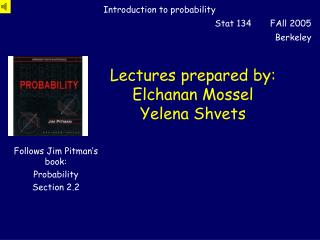DownloadDownload PresentationLectures prepared by: Elchanan Mossel Yelena Shvets

# Lectures prepared by: Elchanan Mossel Yelena Shvets

Download Presentation## Lectures prepared by: Elchanan Mossel Yelena Shvets

- - - - - - - - - - - - - - - - - - - - - - - - - - - E N D - - - - - - - - - - - - - - - - - - - - - - - - - - -
##### Presentation Transcript

1. Lectures prepared by:Elchanan MosselYelena Shvets

2. Binomial Distribution:Mean • Recall: the Mean(m) • of a binomial(n,p) distribution • is given by: m = #Trials £ P(success) = n p • The Mean = Mode (most likely value). • The Mean = “Center of gravity” of the distribution.

3. Standard Deviation • The Standard Deviation (SD) of a distribution, denoted s measures the spread of the distribution. • Def:The Standard Deviation (SD)  of the binomial(n,p) distribution is given by:

4. Example 1: • Let’s compare Bin(100,1/4) to Bin(100,1/2). • 1 = 25, 2 = 50 • 1 = 4.33, 2 = 5 • We expect Bin(100,1/4) to be less spread than Bin(100,1/2). • Indeed, you are more likely to guess the value of Bin(100,1/4) distribution than of Bin(100,1/2) since: Binomial Distribution For p=1/2 : P(50) ¼ 0.079589237; For p=1/4 : P(25) ¼ 0.092132;

5. Histograms for binomial(100, 1/4) & binomial(100, 1/2);

6. Example 2: • Let’s compare Bin(50,1/2) to Bin(100,1/2). • 1 = 25, 2 = 50 • 1 = 3.54, 2 = 5 • We expect Bin(50,1/2) to be less spread than Bin(100,1/2). • Indeed, you are more likely to guess the value of Bin(50,1/2) distribution than of Bin(100,1/2) since: Binomial Distribution For n=100 : P(50) ¼ 0.079589237; For n=50 : P(25) ¼ 0.112556;

7. Histograms for binomial(50, 1/2) & binomial(100,1/2)

8. ,  and the normal curve • We will see today that the • Mean (m) and the • SD (s) give a very good summary of the binom(n,p) distribution via • The Normal Curve with parameters  and .

9. binomial(n,½); n=50,100,250,500 m=25, s=3.54 m=50, s=5 m=125, s=7.91 m=250, s=11.2

10. Normal Curve y m - s m m + s x

11. Standard Normal Standard Normal Density Function: Corresponds to  = 0 and =1

12. Standard Normal Standard Normal Cumulative Distribution Function: • For the normal (,) distribution: • P(a,b) = F((b-m)/s) - F((a-m)/s);

13. Standard Normal

14. Standard Normal -z z

15. Standard Normal Standard Normal Cumulative Distribution Function: • For the normal (,) distribution: • P(a,b) = F((b-m)/s) - F((a-m)/s); • In order to prove this, suffices to show: • P(-1,a) = F((a-m)/s);

16. Change of variables

17. Properties of F: F(0) = 1/2; F(-z) = 1 - F(z); F(-1) = 0; F(1) = 1. does not have a closed form formula!

18. Normal Approximation of a binomial For n independent trials with success probability p: where:

19. binomial(n,½); n=50,100,250,500 m=25, s=3.54 m=50, s=5 m=125, s=7.91 m=250, s=11.2

20. Normal(m,s); m=25, s=3.54 m=50, s=5 m=125, s=7.91 m=250, s=11.2

21. Normal Approximation of a binomial For n independent trials with success probability p: • The 0.5 correction is called the “continuity correction” • It is important when  is small or when a and b are close.

22. Normal Approximation Question: Find P(H>40) in 100 tosses.

23. Normal Approximation to bin(100,1/2). 1-F((40-50)/5) =1-F(-2) = F(2) ¼ 0.9772 What do we get With continuity correction? Exact = 0.971556

24. When does the Normal Approximation fail? bin(1,1/2) m=0.5,s=0.5 N(0.5,0.5)

25. When does the NA fail? bin(100,1/100) m =1, s¼ 1 N(1,1)

26. Rule of Thumb • Normal works better: • The larger  is. • The closer p is to ½.

27. Fluctuation in the number of successes. • From the normal approximation it follows that: • P(m-s to m+s successes in n trials) ¼ 68% • P(m-2s to m+2s successes in n trials) ¼ 95% • P(m-3s to m+3s successes in n trials) ¼ 99.7% • P(m-4s to m+4s successes in n trials) ¼ 99.99%

28. Fluctuation in the proportion of successes. • Typical size of fluctuation in the number of successes is: Typical size of fluctuation in the proportion of successes is:

29. Square Root Law Let n be a large number of independent trials with probability of success p on each. The number of successes will, with high probability, lie in an interval, centered on the mean np, with a width a moderate multiple of . The proportion of successes, will lie in a small interval centered on p, with the width a moderate multiple of 1/ .

30. Law of large numbers Let n be a number of independent trials, with probability p of success on each. For each e > 0; P(|#successes/n – p|< e) ! 1, as n !1

31. Confidence intervals

32. Conf Intervals

33. Conf Intervals is called a 99.99% confidence interval.

34. binomial(n,½); n=50,100,250,500 m=25, s=3.54 m=50, s=5 m=125, s=7.91 m=250, s=11.2

35. Normal(m,s); m=25, s=3.54 m=50, s=5 m=125, s=7.91 m=250, s=11.2

36. bin(50,1/2) + 25 m=50, s =3.535534 bin(50,1/2) m = 25, s = 3.535534 Normal Approximation m=50, s=5 (bin(50,1/2) + 25)* 5/3.535534 m=50, s = 5 bin(100,1/2) m=50, s=5

37. Notre Dame de Rheims

38. Quote Bientôt je pus montrer quelques esquisses. Personnen'y comprit rien. Même ceux qui furent favorables à ma perception des vérités que je voulais ensuite graverdans le temple, me félicitèrent de les avoirdécouvertes au « microscope », quand je m'étais aucontraire servi d'un télescope pour apercevoir deschoses, très petites en effet, mais parce qu'elles étaient situées à une grande distance […]. Là où jecherchais les grandes lois, on m'appelait fouilleur dedétails. (TR, p.346)